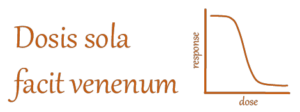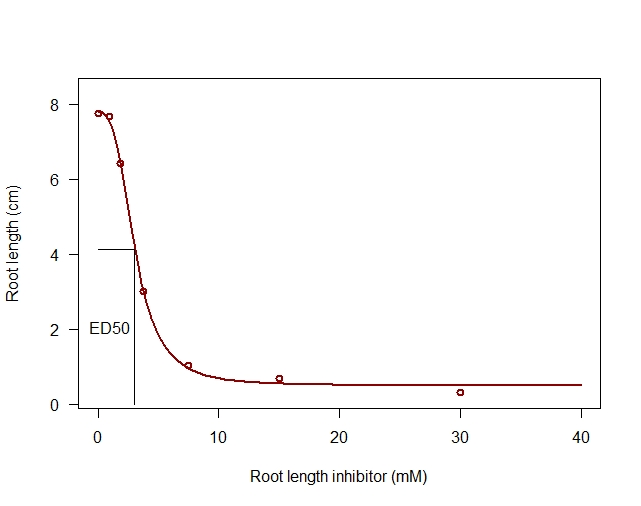# HIDDEN SECRETS

One of the well-hidden secrets of using dose-response bioassays is that you can fine-tune the distribution and the number of doses. You do not need to use the same doses if you want to repeat an experiment, all other things being equal. As long as you get uniformed responses evenly distributed between the upper and lower limits of the response curve to describe the curve’s curvature, you can vary the doses and number of doses used and still compare the results result of fitting and get the toxicity ED50 (Figure 1).Figure 1. One doseresponse curve of the effect of the doses (secanolic acid on Root length of a grass species). The two curves show the same fit, but the change of the x-axis from mere dose to the log(dose) makes it easier to understand the titration concept.

When we use the logarithm of the dose on the x-axis, we get a symmetric curve. Figure 1 shows that there are several ways to show the fit. The most appropriate way is to use the logarithm of the dose to illustrate the curve from virtually no effect to complete kill at large doses.

Although we deal with intact test organisms, we can tentatively explain how a poisonous compound affects the organism’s target enzyme(s). The poisoning is caused by a concentration ratio between the inhibited enzyme and the free enzyme in the organism. In a way, it is a kind of a titration curve that Figure 1 illustrates.